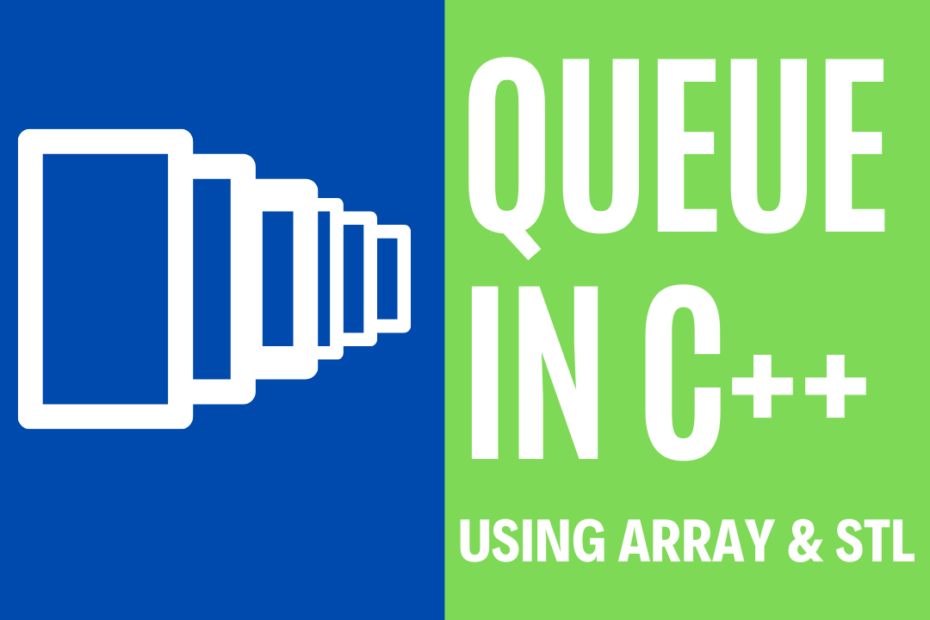# Queue program in C++ using Array and STL

• DSA

The Queue is one of the most important Linear Data Structure, Queue program follows the FIFO rule i.e First In First Out. The contents in a queue exit in the same order they have entered, if an element is to be removed from the queue then the first element that was added will be removed/deleted.

## Operations performed on a Queue

• Q.push() – insert data into the Queue
• Q.pop() – remove data from the Queue
• Q.size() – returns the size of Queue
• Q.front() – displays the front element of the Queue
• Q.back() – displays the back element of the Queue

## Queue Program using Array in C++

``````#include <iostream>
using namespace std;
int queue, front = -1, rear = -1;

void Insert()
{
int val;
if (rear == 100 - 1)
cout << Queue Overflow << endl;
else
{
if (front == -1)
front = 0;
cout << Enter the element to insert in queue :  << endl;
cin >> val;
rear++;
queue[rear] = val;
}
}
void Delete()
{
if (front == -1 || front > rear)
{
cout << Queue Underflow;
return;
}
else
{
cout << Element removed from queue is :  << queue[front] << endl;
front++;
;
}
}

void Display()
{
if (front == -1)
cout << Queue is Empty << endl;
else
{
cout << Elements in queue are : ;
for (int i = front; i <= rear; i++)
cout << queue[i] <<  ;
cout << endl;
}
}

int main()
{
int ch;
cout << 1) Insert to Queue << endl;
cout << 2) Delete from Queue << endl;
cout << 3) Display elements of the Queue << endl;
cout << 4) Exit << endl;
do
{
cout << Enter your choice :  << endl;
cin >> ch;
switch (ch)
{
case 1:
Insert();
break;
case 2:
Delete();
break;
case 3:
Display();
break;
case 4:
cout << Exit << endl;
break;
default:
cout << Invalid Choice << endl;
}
} while (ch != 4);
return 0;
}``````

### Output

``````1) Insert to Queue
2) Delete from Queue
3) Display elements of the Queue
4) Exit
1
Enter the element to insert in queue :
1
1
Enter the element to insert in queue :
2
1
Enter the element to insert in queue :
3
2
Element removed from queue is : 1
3
Elements in queue are : 2 3
4
Exit``````

## Queue Program using STL in C++

``````#include <iostream>
#include <queue>
using namespace std;
//queue program
void Display(queue<int> q)
{
queue<int> dq = q;
while (!dq.empty())
{
cout << dq.front() <<" ";
dq.pop();
}
cout << endl;
}

int main()
{
queue<int> q;
q.push(1);
q.push(2);
q.push(3);

cout <<" Contents in Queue are : ";
Display(q);

cout <<" Front :  "<< q.front() << endl;
cout <<" Back :  "<< q.back() << endl;
cout <<" Size :  "<< q.size() << endl;
cout <<" Popped :  "<< q.front() << endl;
q.pop();
Display(q);
cout <<" FIFO rule First In First Out, 1 was out";
return 0;
}``````

### Output

``````Contents in Queue are : 1 2 3
Front : 1
Back : 3
Size : 3
Popped : 1
2 3
FIFO rule First In First Out, 1 was out``````

This post is part of my #30DaysChallenge to write a blog post every day on what I learn. All the Best ~ Abhiram Reddy